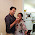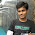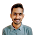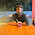To implement this program you need to download Apache POI library and have it in your build path (copy POI jars to lib folder).

Program Flow

1. Get input excel files and get workbook instances
2. Get sheets from those workbooks
3. Get rows from each sheets with same index and compare them
4. Get columns from two selected above rows and compare them

Example

Observe below input files data. Here Row 3 - Column 2 is not matching so the two excel files are not equal.

Expected Output

Comparing Row 0
Cell 0 - Equal
Cell 1 - Equal
Cell 2 - Equal
Cell 3 - Equal
Row 0 - Equal

Comparing Row 1
Cell 0 - Equal
Cell 1 - Equal
Cell 2 - Equal
Cell 3 - Equal
Row 1 - Equal

Comparing Row 2
Cell 0 - Equal
Cell 1 - Equal
Cell 2 - Equal
Cell 3 - Equal
Row 2 - Equal

Comparing Row 3
Cell 0 - Equal
Cell 1 - Equal
Row 3 - Not Equal

The two excel sheets are Not Equal
Cell 2 - NOt Equal

Java Code

import java.io.File;
import java.io.FileInputStream;
import org.apache.poi.hssf.usermodel.HSSFCell;
import org.apache.poi.xssf.usermodel.XSSFCell;
import org.apache.poi.xssf.usermodel.XSSFRow;
import org.apache.poi.xssf.usermodel.XSSFSheet;
import org.apache.poi.xssf.usermodel.XSSFWorkbook;

public class CompareExcel {

public static void main(String[] args) {
try {
// get input excel files
FileInputStream excellFile1 = new FileInputStream(new File(
"C:\\sheet1.xlsx"));
FileInputStream excellFile2 = new FileInputStream(new File(
"C:\\sheet2.xlsx"));

// Create Workbook instance holding reference to .xlsx file
XSSFWorkbook workbook1 = new XSSFWorkbook(excellFile1);
XSSFWorkbook workbook2 = new XSSFWorkbook(excellFile2);

// Get first/desired sheet from the workbook
XSSFSheet sheet1 = workbook1.getSheetAt(0);
XSSFSheet sheet2 = workbook2.getSheetAt(0);

// Compare sheets
if(compareTwoSheets(sheet1, sheet2)) {
System.out.println("\n\nThe two excel sheets are Equal");
} else {
System.out.println("\n\nThe two excel sheets are Not Equal");
}

//close files
excellFile1.close();
excellFile2.close();

} catch (Exception e) {
e.printStackTrace();
}

}

// Compare Two Sheets
public static boolean compareTwoSheets(XSSFSheet sheet1, XSSFSheet sheet2) {
int firstRow1 = sheet1.getFirstRowNum();
int lastRow1 = sheet1.getLastRowNum();
boolean equalSheets = true;
for(int i=firstRow1; i <= lastRow1; i++) {

System.out.println("\n\nComparing Row "+i);

XSSFRow row1 = sheet1.getRow(i);
XSSFRow row2 = sheet2.getRow(i);
if(!compareTwoRows(row1, row2)) {
equalSheets = false;
System.out.println("Row "+i+" - Not Equal");
break;
} else {
System.out.println("Row "+i+" - Equal");
}
}
return equalSheets;
}

// Compare Two Rows
public static boolean compareTwoRows(XSSFRow row1, XSSFRow row2) {
if((row1 == null) && (row2 == null)) {
return true;
} else if((row1 == null) || (row2 == null)) {
return false;
}

int firstCell1 = row1.getFirstCellNum();
int lastCell1 = row1.getLastCellNum();
boolean equalRows = true;

// Compare all cells in a row
for(int i=firstCell1; i <= lastCell1; i++) {
XSSFCell cell1 = row1.getCell(i);
XSSFCell cell2 = row2.getCell(i);
if(!compareTwoCells(cell1, cell2)) {
equalRows = false;
System.err.println("       Cell "+i+" - NOt Equal");
break;
} else {
System.out.println("       Cell "+i+" - Equal");
}
}
return equalRows;
}

// Compare Two Cells
public static boolean compareTwoCells(XSSFCell cell1, XSSFCell cell2) {
if((cell1 == null) && (cell2 == null)) {
return true;
} else if((cell1 == null) || (cell2 == null)) {
return false;
}

boolean equalCells = false;
int type1 = cell1.getCellType();
int type2 = cell2.getCellType();
if (type1 == type2) {
if (cell1.getCellStyle().equals(cell2.getCellStyle())) {
// Compare cells based on its type
switch (cell1.getCellType()) {
case HSSFCell.CELL_TYPE_FORMULA:
if (cell1.getCellFormula().equals(cell2.getCellFormula())) {
equalCells = true;
}
break;
case HSSFCell.CELL_TYPE_NUMERIC:
if (cell1.getNumericCellValue() == cell2
.getNumericCellValue()) {
equalCells = true;
}
break;
case HSSFCell.CELL_TYPE_STRING:
if (cell1.getStringCellValue().equals(cell2
.getStringCellValue())) {
equalCells = true;
}
break;
case HSSFCell.CELL_TYPE_BLANK:
if (cell2.getCellType() == HSSFCell.CELL_TYPE_BLANK) {
equalCells = true;
}
break;
case HSSFCell.CELL_TYPE_BOOLEAN:
if (cell1.getBooleanCellValue() == cell2
.getBooleanCellValue()) {
equalCells = true;
}
break;
case HSSFCell.CELL_TYPE_ERROR:
if (cell1.getErrorCellValue() == cell2.getErrorCellValue()) {
equalCells = true;
}
break;
default:
if (cell1.getStringCellValue().equals(
cell2.getStringCellValue())) {
equalCells = true;
}
break;
}
} else {
return false;
}
} else {
return false;
}
return equalCells;
}
}

1.This Example code is very usefully....Thanks

2.Is this working? I got this error - at CompareExcel.main(CompareExcel.java:20)
Caused by: java.lang.ClassNotFoundException: org.apache.xmlbeans.XmlObject

1.You might have missed the XmlBeans jar file

3.Can you please provide me the steps, hot to execute the above code in eclipse

1.I have shared the eclipse project, download it and import to eclipse

2.Hi, this same code workable eclipse?

4.Desari Srinivas, is this project available for reuse? That is, do I have permission to use it and/or edit it?
Thanks

1.5.Thanks you Srinivas.... This code helped me a lot !!!!!!!

6.Can you please tell me how to return the entire row if there is a mismatch in a particular cell in that row ?

1.Instead of returning equalRows boolean value, return row1 and row2 in a arraylist

7.hiiii Dasari u didnt answered of my post one error was coming and you also deleted it ...is some thing i did wrong so i apologiges...
thanku u :-)

1.8.hi srinivas, i have a similar requirement with small change. Requirement is that "have to compare two rows from sheet1 and sheet2 but it should not be in order. Means if i say 1st row in sheet 1, presents in sheet2 as row 10 but both are equal".
so here comparison logic would be take 1st row from sheet1 -> compare with all the rows in sheet2 one by one until its get matched. if matches then say row1 is matched or say failed. Can you help me here, Please. Thanks in advance.

1.Hi
I have a similar requirement.
Can anyone help me please

9.How to compare files in csv format , file to file, sheet to sheet etc

10.Hi Srinivas, i have a similar requirement with small change. Requirement is that "have to compare two rows from sheet1 and sheet2 but it should not be in order. Means if i say 1st row in sheet 1, presents in sheet2 as row 10 but both are equal".
so here comparison logic would be take 1st row from sheet1 -> compare with all the rows in sheet2 one by one until its get matched. if matches then say we need to change the Font of matched row to GREEN in anyone excel sheet and if it does not matches then change Font to RED in the same excel sheet.Can you help me here, Please. Thanks in advance.

11.I`ll use it because I like
comparable vs comparator java https://explainjava.com/comparable-comparator-java/ and I'm used to working this way. The script needs to be compiled, and yes,
I see some conditions. To understand the algorithm, I found several tutorials, perhaps this will be very useful for those who do not finally understand the sorting, and how I search for explanations.

12.This comment has been removed by the author.

13.This comment has been removed by the author.

14.getCellType is deprecated .How do I modify it?

15.im trying to implement extent report for the above code. im getting error when i put logstatu.PASS in else condition in comareTwoCells method.i m able to capture the failed report in extent report under if(!compareTwoCells(cell1, cell2) but not in else case- getting nullPointer Exception. if i remove logstatus.PASS statement from else block -- it works. Please help how to log the pass report here

16.Compare two excels files and if we find any differences in one excel sheet that difference or row should be added in a given excel path and after adding the differences in that excel it should get highlighted ? Is it possible to add automatically ?

17.hi srinivas, i have similar requirmenet but need some more add on,
Like if any of the cell is not matched or mismatched , i need to Write into the one of the excel write that the cell is not matched in last of the row

18.how to take comparison result in excel

BlogrollSrinivas Dasari find me on facebook follow me on twitter